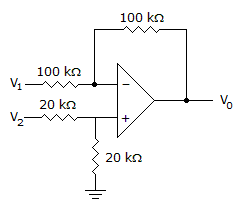# Electronic Devices - Op-Amp Applications - Discussion

Discussion Forum : Op-Amp Applications - General Questions (Q.No. 31)
31.
Determine the output voltage when V1 = –V2 = –1 V.0 V
–2 V
1 V
2 V
Explanation:
No answer description is available. Let's discuss.
Discussion:
6 comments Page 1 of 1.

RUSEN CAVUSOGLU said:   8 years ago
V1 =-1(-V2=-1) mean is V2 = 1 and result should be Vout = +2Volt.

I think answers or Question is wrong.

Vout1 = -1v*(-1/1)
Vout2 = V2*(20/20+20)*1+(100/100).

= 1V Vout2 = 1*0.5*2.

Vout2 = 1V.
Vout = Vout1+Vout2.
Vout = 1V+1V.
Vout = 2V.

M. Hema Narayana said:   9 years ago
I think the answer is 2.

Because of that if we apply superposition then,

v01 = -v1 v02 = v2.

-v1+v2 = -(-1)+1 = 2.

Surendra Bansode said:   9 years ago
I think Vd = V1-V2.

Hence Vo = 2V.

Sahal said:   7 years ago
Vo = Rf/R1 (V2 - V1).
= 100/100 (1 - (-1)).
= 1(1 + 1).
= 2 V.

Md saquib said:   6 years ago
(-rf/ri) * v1 = 1,
(r2/r2+r1) = 1/2 * (1+rf/r1 = 3,
So, v2-v1 = 3 - 1 = 2v.

Siva said:   8 years ago
Vo = V2 - V1.

Vo = 2.